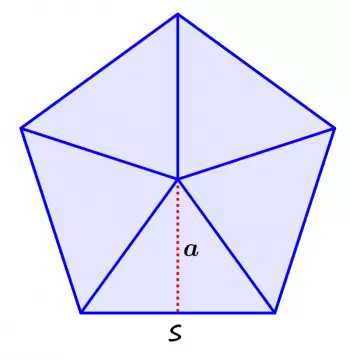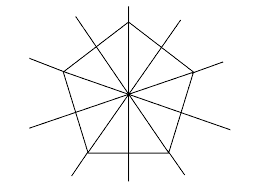# Pentagon Shape: Area, Perimeter, and Lines of SymmetryAn outstanding figure in geometry is the pentagon. It is usual that, for various reasons, we need to find the area of ​​the pentagon.

Obtaining the area and perimeter of a pentagon is easy. First, however, we must know some basic characteristics, such as how many sides a pentagon has (five) or how many diagonals a pentagon have (five).

One of the most recognizable buildings in the world with this shape is The Pentagon. It is the headquarters of the United States Department of Defense. The Pentagon is a five-sided building with a perimeter of 2,611 feet (796 meters).

## What Is a Pentagon Shape?

A pentagon shape is a polygon with five sides and five vertices.

A polygon is a two-dimensional geometric figure with a finite number of non-collinear consecutive line segments connected by their two ends, forming a closed space.

These five-sided-polygons can be classified into several categories, depending on their shape:

• Regular pentagon: It is the one that has all its sides of equal length, and all angles are equal.

• Irregular pentagon: It does not have all its sides and angles equal.

• Convex pentagon: All interior angles are less than 180°.

• Concave pentagon: At least one of the interior angles is greater than 180°.

## How Many Diagonals Does a Pentagon Have?A diagonal is a line or segment that joins one vertex to another within the same shape. The number of diagonals in a pentagon shape is five.

## How Many Lines of Symmetry Does a Pentagon Have

A pentagon has five axes of symmetry in a regular polygon.In geometry, axes of symmetry are imaginary lines dividing the pentagon shape into equal parts so that each polygon has a certain number of axes of symmetry. Some geometric figures have only one axis, while others have two or even infinity, as with the circle or the oval.

The pentagon lines of symmetry are drawn from each middle point of its edges to the furthest vertex. As the number of sides of this regular polygon is equal to five, a pentagon has five symmetry lines.

## What Are the Pentagon Properties?

Below we list the most significant characteristics of this type of flat shape:

• A pentagon is made up of five sides and five angles. The different types of pentagons are classified based on their sides and angles.

• All five interior angles add up to 540º.

• If it is regular, all interior angles measure 108°, and therefore all exterior angles measure 72°.

• The central angle of the regular pentagon 360° / 5 = 72º. The central angle is formed by two straight lines that join the two ends of a side to the center of the figure.

• A pentagon shape is composed of five diagonals.

• From each vertex, two diagonals can be drawn to another vertex.

• When the pentagon is regular, the diagonals at each vertex form three 36° angles and divide the regular pentagon into three isosceles triangles.

• The perimeter of a regular or irregular pentagon is the sum of the lengths of all its sides. If it is a regular polygon, it is the length of one side multiplied by five.

## How Can You Find the Area of ​​a Pentagon?

There are several ways to obtain the area of ​​a regular pentagon. An area pentagon formula is:

A = (5/2)·c·a

Where "c" refers to the length of a side, "a" refers to the size of the apothem of the pentagon.

If we know the perimeter: the formula becomes A = p·a / 2; where "p" is the perimeter.

If we know the apothem, we can use the formula:

A = (5 · (a2)) / (4 · tan(36))

In the formula, "a" refers to the apothem of the shape.

### Calculating the Area of ​​a Triangle

The area of ​​a pentagon can also be calculated by dividing it into triangles:

If we draw two diagonals, from vertex to vertex, without intersecting, three isosceles triangles are formed. The sum of the area of ​​these triangles is equal to the area of ​​the pentagon.

If we join each vertex to the center of the geometric figure, we will have five equilateral triangles left. We will also obtain the total surface with the sum of the surface of these five triangles.

Author:

Published: October 1, 2022
Last review: October 1, 2022